# Assumptions and limitations of IRR and NPV

Discuss the assumptions and limitations of IRR and NPV and select which method is most superior and beneficial for an organization.

This post was part of TyroCity discussion forum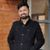Angel Paudel

IRR (Internal Rate of Return) can be defined as the discount rate at which the NPV value of the project is equal to 0. If the IRR is higher than the required return of rate, this often means that the project will have a profitable outcome. It is a relative measure and is expressed in percentage value. This method helps in understand the return of the project and is thus commonly used by the business manager.

NPV (Net Present Value) is calculated by subtracting the present value of cash outflow from the present value of cash inflow. It gives the return in currency which the company expects to make out of the project. This method helps in effective decision making for projects with changes in cash flow (Martin, 2018). As the expression is in the form of currency, this is better understood by any people in general.

IRR assumes that all the future cash flow during the project lifetime is reinvested into the project while earning the same IRR over the remaining life of the project. IRR moves money back into the past instead of future with this method so this method is neither realistic nor feasible. This could give inaccurate outcome for projects and a different outcome in the reality. IRR also doesn’t account for the additional shareholder wealth while doing a calculation for the profitability of the project (Dudley, 1972). Another assumption it makes is that any project would require the same amount of investment and based on this itself the project with the highest IRR is considered to be the best one.

NPV, on the other hand, takes into consideration the time value of money. The method assumes that the reinvestment rate is equal to the cost of capital while future cash flows are discounted at the cost of capital (Beaves, 1988). NPV makes a different assumption which is that it is reinvested but at the required rate of return. It takes into account the additional wealth that the stakeholder accommodates while calculating the project’s profitability. NPV assumes that the discount rate is unchanged during the project lifeline as with a different discount rate, the project would have multiple NPV values. It also assumes that the investment is instantly made once the cash flow is recovered. With NPV, the decision to accept a project can’t be revisited meaning if the project is taken today based on it giving positive cash flow but a year down the line once the project shows a negative return to a point the company is better off abandoning the project, traditional NPV assumes that these kinds of outcome isn’t possible.

If a project has both positive and negative cash flow is lots of changes, it gives multiple IRR for the same project. This makes it impossible to select a project simply based on IRR. The result/outcome is displayed in percentage value which is also deceiving. For example, if a project with has an IRR value of 20% for the project with an initial investment of NRs. 100, and the other project just has an IRR value of 15% but with an initial investment of NRs. 10,000. The first project should be selected as per the calculation of IRR but if we look at the value generation, the second one is a lot higher. IRR not accounting for the discount rate changes makes it even less suitable for long-term projects.

NPV fails into taking account the resources that are required to implement the project. It also fails to take into account the risk related to discount rate as the discount rate of today can very well be different during any year of the project lifetime. NPV calculations require us to calculate the future cash flow, most of which are unknown. No matter how well one calculates this, there’s always uncertainty in cash flow forecast further points back at uncertainty in NPV.

Taking into account what NPV and IRR have to offer along with the assumptions and limitations they stand on for the evaluation of two or more mutually exclusive project, it’s better to go with NPV over IRR. As IRR gives multiple values during the lifetime of the project and makes the selection process further complicated whereas NPV makes a realistic assumption (comparatively) and gives a better measure of profitability.

References

Beaves, R. (1988). Net Present Value and Rate of Return: Implicit and Explicit Reinvestment Assumptions. The Engineering Economist , 33 (4), 275-278.

Dudley, C. (1972). A Note on Reinvestment Assumptions in Choosing between Net Present Value and Internal Rate of Return. The Journal Of Finance , 27 (4), 907.

Martin, Y. (2018). Value Creation Mechanics: An Analytical Reduction of Cash Flows and Net Present Value to Accounting. SSRN Electronic Journal , 3-4.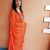Net Present Value (NPV)

NPV technique is a discounted cash flow method that considers the time value of money in evaluating capital investments. It is a method of calculating return on investment of a project by taking into consideration all the expected cash flows from the project and translating those into today’s rupees value (Gallo, 2014). In simple term, NPV is the difference between the present value of future cash inflows and initial investment. It represents the contribution of the investment to the value of the firm and the wealth of shareholders (Moyer, McGuigan, Rao, & Kretlow, 2012). The proposed project is selected if the NPV is equal to or greater than zero.

Assumptions
The NPV of a project is calculated based on the following assumptions:

• A rupee in future will not worth as much as a rupee today. So all the future cash flows are translated to present value by discounting them at the cost of capital.
• The inflow or outflow of cash other than the initial investment occur at the end of each period.
• The discount rate or cost of capital remains same throughout the life of the project.
• The cash generated by a project is immediately reinvested at the cost of capital.

Limitations

Some of the limitations of this method are:

• The assumption about the immediate reinvestment of cash generated by the project may not be always reasonable due to changing economic conditions (Accounting for Management.Org 14).
• It does not consider the non-cash benefits generated from a project into consideration while making decisions.
• Since NPV is calculated on the basis of estimated cash flows and discount rate, there is room for error and accuracy cannot guaranteed.
• The cost of capital may change over the life of period and NPV does not provide criteria for addressing the change in discount rate.

Internal Rate of Return (IRR)

IRR is the estimated rate of return offered by an investment that makes the present value of subsequent net cash inflows equal to the initial investment. It is the rate of return at which NPV of the investment will be zero. Thus it is the break even rate that equates the present value of cash inflows with present value of cash outflows (Gallo, A Refresher on Internal Rate of Return, 2016). Based on this criteria, the projects having IRR higher than the required rate of return are accepted.

Assumptions

The assumptions of IRR is similar to that of NPV except for the reinvestment rate of generated cash flow. It includes:

• It considers both the magnitude and timing of cash flows.
• The discount rate does not change over the life of the project.
• The cash flows generated from the proposed project are reinvested at the calculated IRR.

Limitations

Some of the major limitations of IRR method are:

• It does not address issues of scale or size of investment. It means if the IRR of a project is estimated to be 20%, it may be 20% of Rs. 100, Rs. 1000 or Rs. 100000.
• IRR alone is not sufficient for decision making process. It need to be compared with the required rate of return.
• The reinvestment of the generated cash flows at IRR is an unrealistic assumption. To generate the return equal to IRR, the firm should have multiple investment opportunities that is not always true in real market scenario.
• There is chance of getting multiple IRRs in case of projects that require additional investment during the life time of the project or projects with unconventional cash flows that complicates the decision making process.

Which is Better: NPV or IRR

NPV and IRR are recurrently used methods for evaluating projects. In case of independent projects, the NPV and IRR both give similar results. The NPV is positive if the IRR is greater than cost of capital and vice-versa. But, in case of mutually exclusive projects, they might give contradictory results. Both NPV and IRR have their own significance but if we have to choose between these two, NPV is superior and beneficial because of the following reasons:

• NPV assumes that the cash flows are reinvested at cost of capital which is more realistic and practical than the assumption of IRR where reinvestment rate is equal to the calculated IRR.
• NPV shows the value addition to the company in rupee value which is easier to understand than the straight forward calculations shown in percentage by IRR. The actual amount by which the project adds value to the firm cannot be estimated easily by using IRR.

References

Accounting for Management.Org. (n.d.). Net Present Value Method. Retrieved from Capital Budgeting Techniques

Gallo, A. (2014, November 19). A Refresher on Net Present Value. Hravard Business Review .

Gallo, A. (2016, March 17). A Refresher on Internal Rate of Return. Harvard Business Review .

Moyer, R. C., McGuigan, J. R., Rao, R., & Kretlow, W. J. (2012). Contemporary Financial Management (12th ed.). Oklahoma: Cengage Learning.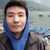Internal Rate of Return (IRR) is the discount rate that equates the NPV equivalent to zero. It helps in the decision making for selection of project as higher the IRR than the cost of capital positive signal for the selection of the project. (Moyer, McGuigan, Rao, & Kretlow, 2012)

Assumption of IRR

IRR is the discounted rate of also called an interest rate. This helps investors decide on which investment yields higher return. But on of its biggest assumption is that the cash flow generated each year is reinvested in the same project and its rate of return is the IRR. This may not be realistic as people may invest in other projects and the actual rate of return may be lower than the assumed IRR.

Limitation of IRR

IRR is basically just the interest rate that is used to calculate how much the project will earn at the end of the period when cash flow of each year is reinvested. However with varied cash flow which may be positive and negative in some years can generate multiple IRRs. This will make it impossible to choose which IRR to follow.

Net Present Value (NPV) is a tool used in capital budgeting for decision making of either selecting or rejecting a project. It calculates the positive or negative return from investment in the allocated period by calculating the discounted cash flows.

"The net present value–that is, the present value of the expected future cash flows minus the initial outlay–of an investment made by a firm represents the contribution of that investment to the value of the firm and, accordingly, to the wealth of the firm’s shareholders (Moyer, McGuigan, Rao, & Kretlow, 2012).”

Assumption of NPV

The discount rate at which NPV is calculated is assumed. We know that lower discount rate yield positive NPV while higher NPV yield negative and since we need positive NPV to select a project there is no concrete way to select a discount rate other than assuming.

Limitation of NPV

NPV only calculates up to the point of positive cash flow and does not review the profit made by the project. This is a limitation. As after the breakeven the cash flow goes negative in the NPV profile.

Reference

Moyer, C. R., McGuigan, J. R., Rao, R., & Kretlow, W. J. (2012). Contemporary Financial Management. Natorp Boulevard: South-Western, Cengage Learning.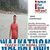Both NPV and IRR are considered scientific techniques of a project’s financial appraisal and both are commonly used. The NPV is an absolute value of a gain or loss, while IRR is a rate of return from a given investment and, therefore, more appropriate for comparison between different project proposals as well as between a given IRR and different costs of capital. In this respect IRR seems to be having an advantage (Osborne, 2010). This, however, may not always be so.

The biggest problem with IRR is that it is not uniquely defined and we may get more than one IRR in some cases.

Let us consider the following cash flow

End of year Net Cash Flow
0 -1000
1 +2300
2 -1320

From the above cash flow pattern, we find IRR =10% and 20%, but actually both of them may not be correct. In such a situation, we may compel to abandon and the IRR and use Net Present Value criteria to make decision.

Another problem in using IRR is that it does not distinguish between lending and borrowing. For example, in following illustration we have two projects A and B, with their cash flows as

For Example,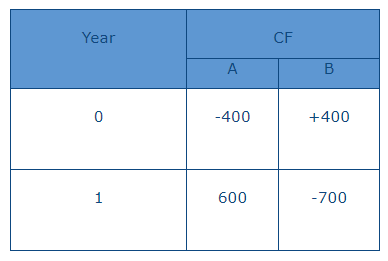Project A is lending (investing) project while project B is borrowing (financing) project. The NPV of A and B at 10% discount rate is

A = - 400 + 600/ (1.1) = - 400 + 545.45 = 145.45

B = +400 - 700/ (1.1) = + 400 - 636.36 = -236.36

If we calculate IRR of these proposals it is 50% and 75% respectively, indicating that the project B is better. The reality is that A has a positive NPV while B is having negative NPV.

Using NPV and IRR may again create problems, when the patterns of each cash flows is different. For example: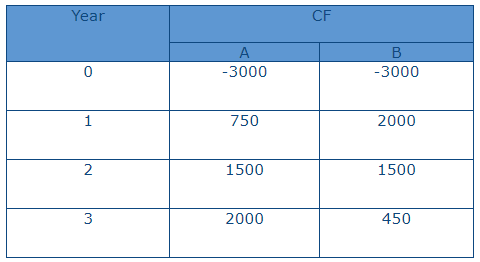NPV at 10% for A is 424.11 while for B it is Rs. 395.94. Thus, A gives a higher NPV. However if we calculate IRR for the two projects, it is 16.75% and 19.18% respectively for projects A and B. This creates a dilemma.

The conflict between the two techniques is mainly due to the differences in the timings of the cash-flows. In project A higher amounts are occurring in the later years of the projects and its NPV will fall faster in respect of increase in discount rates. In project B the major cash flows occur initially hence its rate of fall is slower.

A comparison between NPV, and IRR shows that NPV is the best capital budgeting technique because of its conceptual charity and methodology of calculation.

For Example,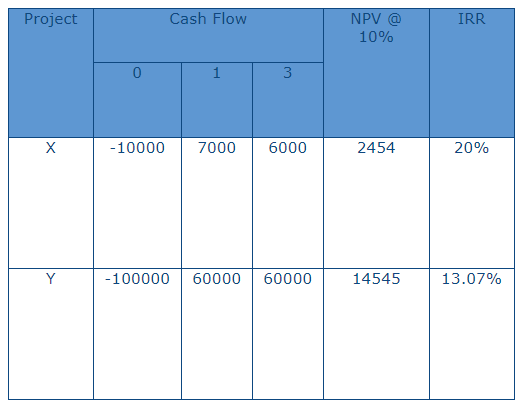In the above case project X seems to be superior both in respect to IRR, while project Y is better if we go by NPV. In such a situation, decision should be taken on the basis of NPV, (even if other projects have a higher rate of return). This is because the objective of firm is wealth maximization. Project Y creates extra wealth of Rs. 14545 while project X creates wealth of Rs. 2454 only. Hence, NPV rule is always the best when we have to make decision about a specific project or number of projects and when fund constraint is no problem, i.e. funds can be obtained from the market by paying its cost. However, when funds availability is a constraint, i.e. limited funds are available and we have to choose among different alternatives, then obviously NPV cannot work.

References

Osborne, M. (2010). A resolution to the NPV-IRR debate? Quarterly Review of Economics and Finance, 50 (2), ISSN 10629769. , 234-239.